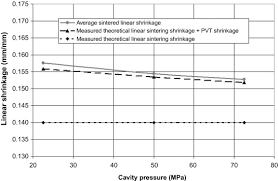## How to Calculate and Solve for Part Shrinkage Ratio | Polymer & TextileThe image above represents part shrinkage ratio.

To compute for part shrinkage ratio, two essential parameters are needed and these parameters are mold dimension (DM) and part dimension (DP).

The formula for calculating part shrinkage ratio:

RPS = DM – DP / DM

Where:

RPS = Part Shrinkage Ratio
DM = Mold Dimension
DP = Part Dimension

Let’s solve an example;
Find the part shrinkage ratio when the mold dimension is 8 and the part dimension is 10.

This implies that;

DM = Mold Dimension = 8
DP = Part Dimension = 10

RPS = DM – DP / DM
RPS = 8 – 10 / 8
RPS = -2 / 8
RPS = -0.25

Therefore, the part shrinkage ratio is -0.25.